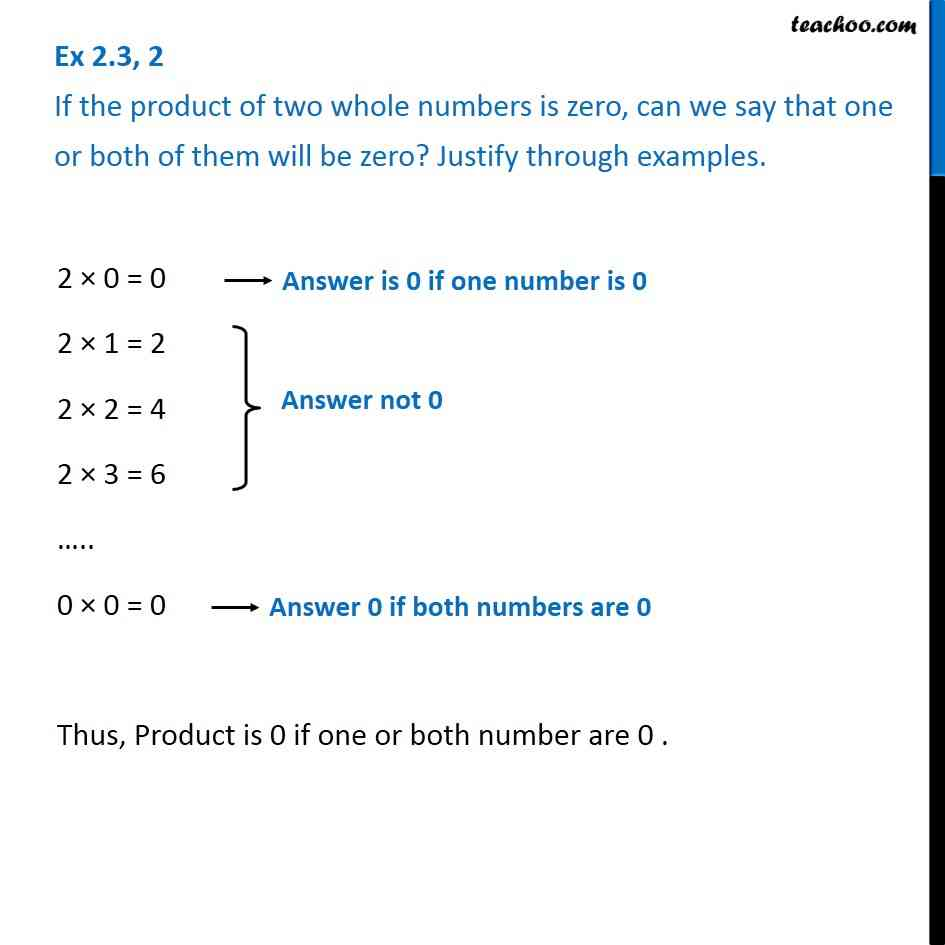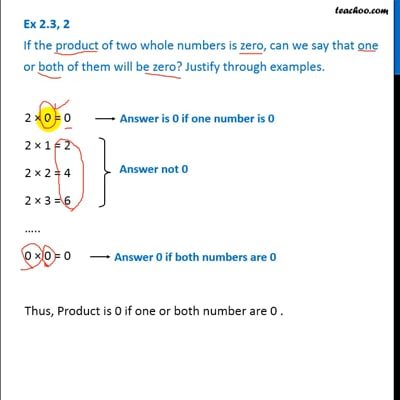Ex 2.3

Chapter 2 Class 6 Whole Numbers
Serial order wiseThis video is only available for Teachoo black users

### Transcript

Ex 2.3, 2 If the product of two whole numbers is zero, can we say that one or both of them will be zero? Justify through examples. 2 × 0 = 0 -> Answer is 0 if one number is 0 2 × 1 = 2 2 × 2 = 4 2 × 3 = 6 ….. 0 × 0 = 0 -> Answer is 0 if both numbers are 0 Thus, Product is 0 if one or both number are 0 .